Learning Objective

In this lesson we will learn about the main subatomic particles which make up atoms.

Learning Outcomes

By the end of this lesson you will be able to:

• Describe the properties of protons, neutrons and electrons.

• Describe the arrangement of protons, neutrons and electrons in an atom.

• Describe the distribution of mass and charge within an atom.

BUY THE YEAR 9 CHEMISTRY WORKBOOK

## Introduction

• Atoms are composed of subatomic particles called protons, neutrons and electrons.
• The protons and neutrons form a cluster at the centre of the atom, called the nucleus.
• The electrons move around the nucleus at high speeds, forming an electron cloud.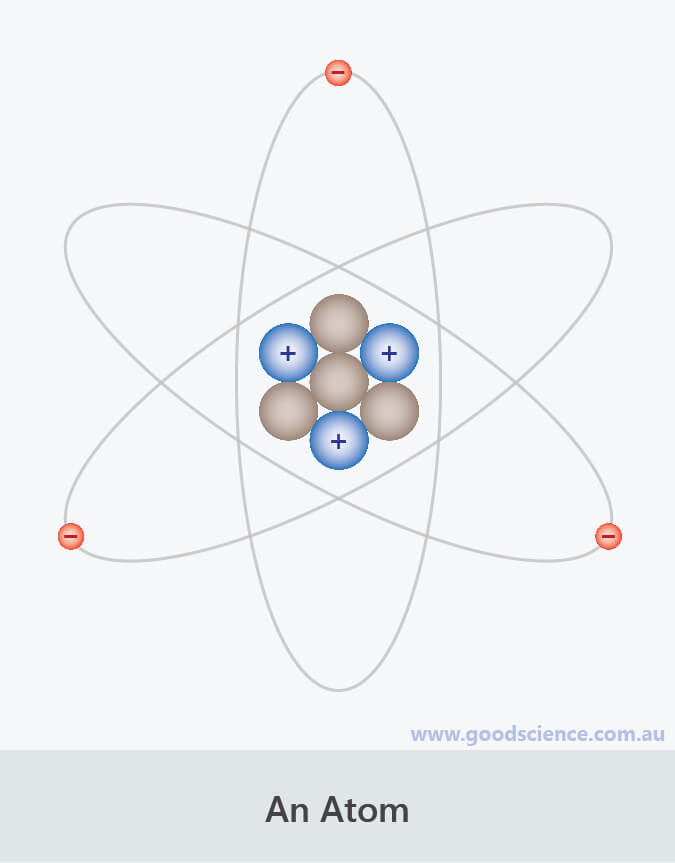Atoms are composed of protons, neutrons and electrons.

## Protons

• Protons are subatomic particles with a positive charge (+1).
• Protons have a mass of 1 atomic mass unit.
• The number of protons in an atom determines the type of atom.
Examples:
If an atom has 7 protons, it is a nitrogen atom.
If an atom has 16 protons, it is a sulfur atom.
If an atom has 47 protons, it is a silver atom.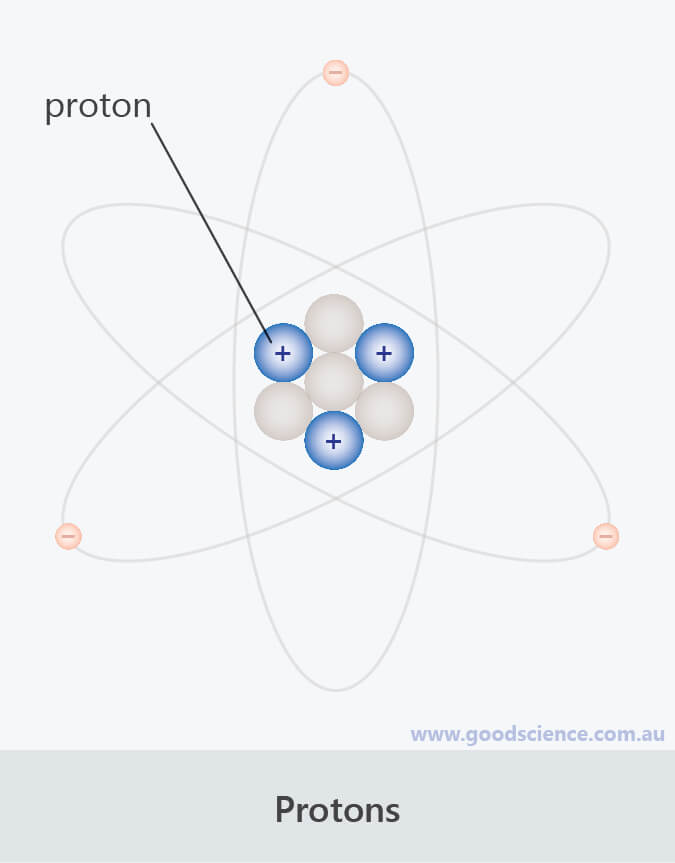Protons have a mass of 1 atomic mass unit and a positive charge.

## Neutrons

• Neutrons are subatomic particles with no charge; in other words, they are neutral.
• Neutrons have a mass of 1 atomic mass unit.
• The number of neutrons in a particular type of atom can vary.
Examples:
99% of carbon atoms have 6 neutrons, 1% have 7 neutrons.
76% of chlorine atoms have 18 neutrons, 24% have 20 neutrons.
79% of magnesium atoms have 12 neutrons, 10% have 13 neutrons, 11% have 14 neutrons.
• The number of neutrons in an atom is similar to the number of protons, but as the size of atoms increases, the proportion of neutrons gradually increases.
For example, small atoms, such as helium, oxygen and neon, have a proton:neutron ratio of approximately 1:1, whereas large atoms, such as gold, mercury and lead, have a proton:neutron ratio of approximately 1:1.5.
This is because, as the nuclei of atoms become larger, more neutrons are required to stabilise them.Neutrons have a mass of 1 atomic mass unit and no charge.

## Electrons

• Electrons are subatomic particles with a negative charge (–1).
• Electrons are much smaller than protons and neutrons.
They have a mass of approximately 1/1800 of an atomic mass unit.
Therefore, almost all of the mass of an atom is in the nucleus.
• The number of electrons in an atom is the same as the number of protons.
Therefore, although atoms contain charged particles, they have no overall charge.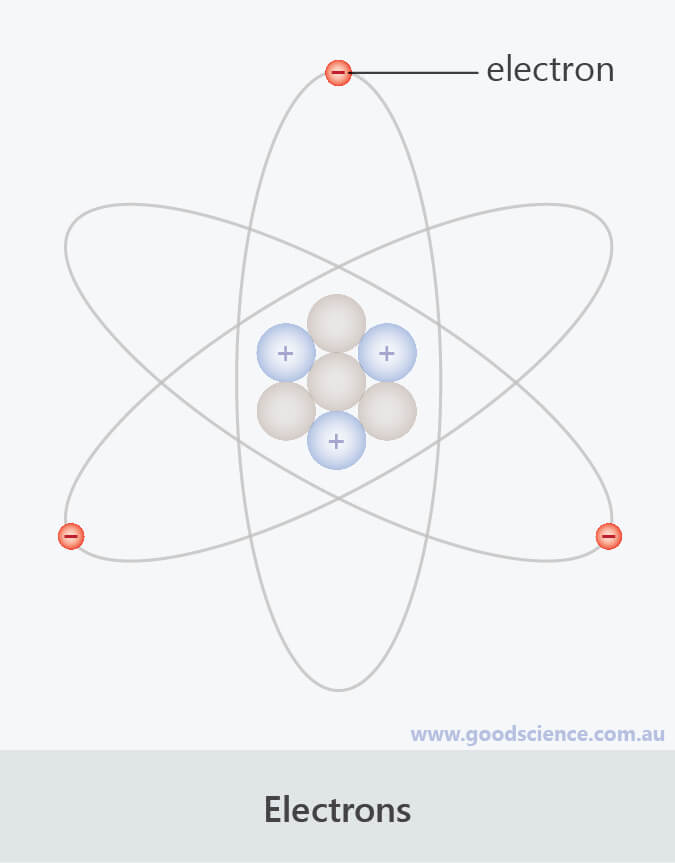Electrons have a mass of 1/1800 of an atomic mass unit and a negative charge.

## Nuclei

• Since protons are positive and neutrons are neutral, the overall charge of a nucleus is positive.
• The nucleus is extremely dense.
It accounts for 99.9% of the mass of an atom but takes up less than 0.001% of the volume.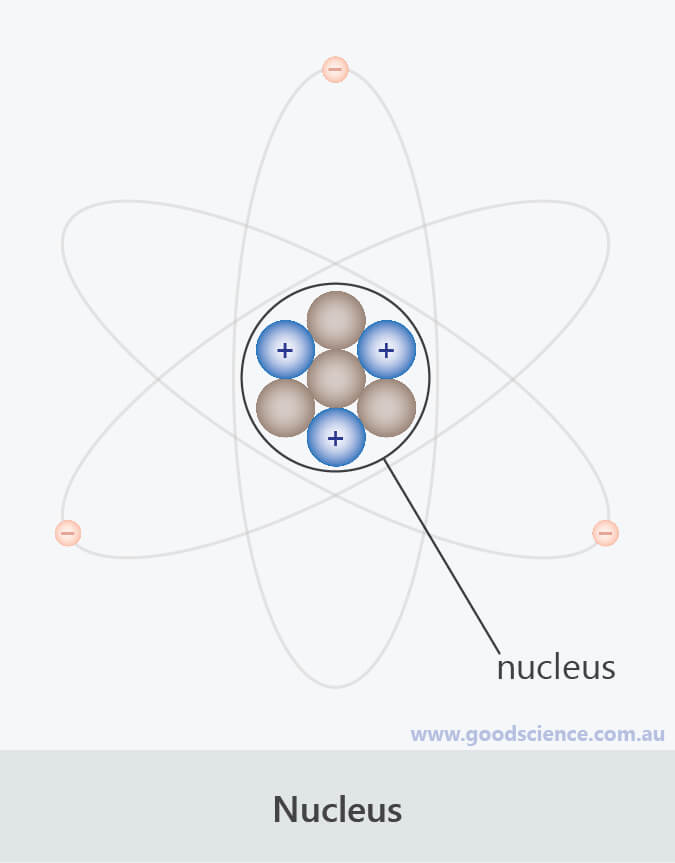Protons and neutrons form a dense cluster at the centre of an atom, called a nucleus.

## Summary

• Atoms are composed of subatomic particles called protons, neutrons and electrons.
• Protons have a mass of 1 atomic mass unit and a positive charge (+1).
The number of protons determines the type of atom.
• Neutrons have a mass of 1 atomic mass unit and no charge.
• Electrons have a mass of 1/1800 of an atomic mass unit and a negative charge (–1).
• Protons and neutrons form a dense cluster at the centre of an atom, called a nucleus.
• Electrons move around the nucleus, forming an electron cloud.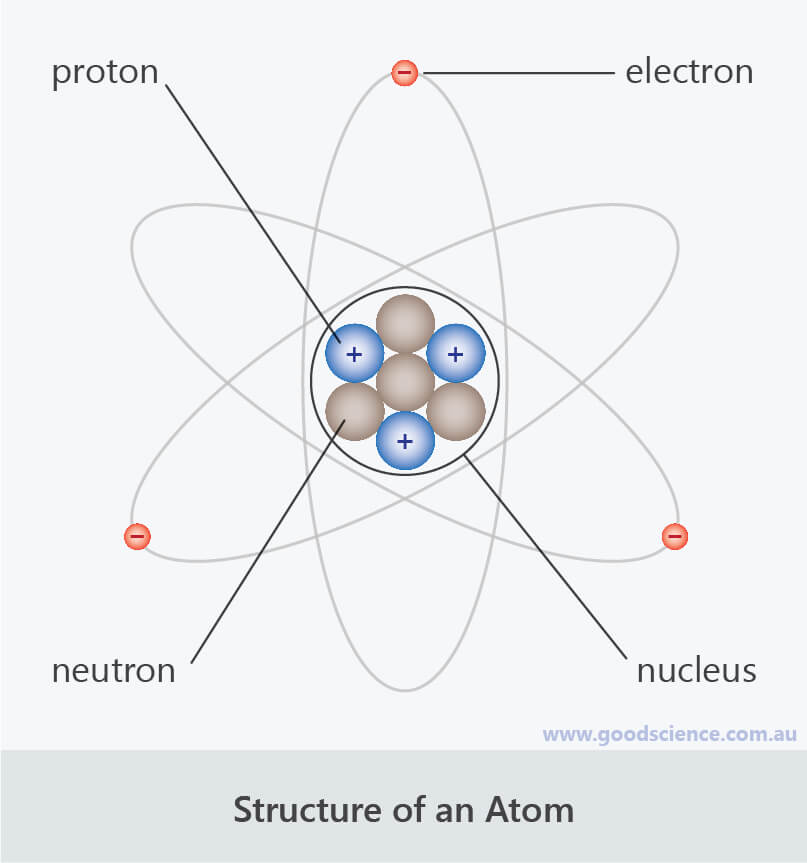Particle / Structure Location Charge Mass (atomic mass units) Proton Nucleus +1 1 Neutron Nucleus 0 1 Electron Electron Cloud –1 1/1800 Nucleus Centre of atom positive (amount depends on atom) (depends on atom) Electron Cloud Around the nucleus negative (amount depends on atom) (depends on atom, but negligible) Atom – 0 (depends on atom, approximately same as nucleus)

Summary of atomic structure